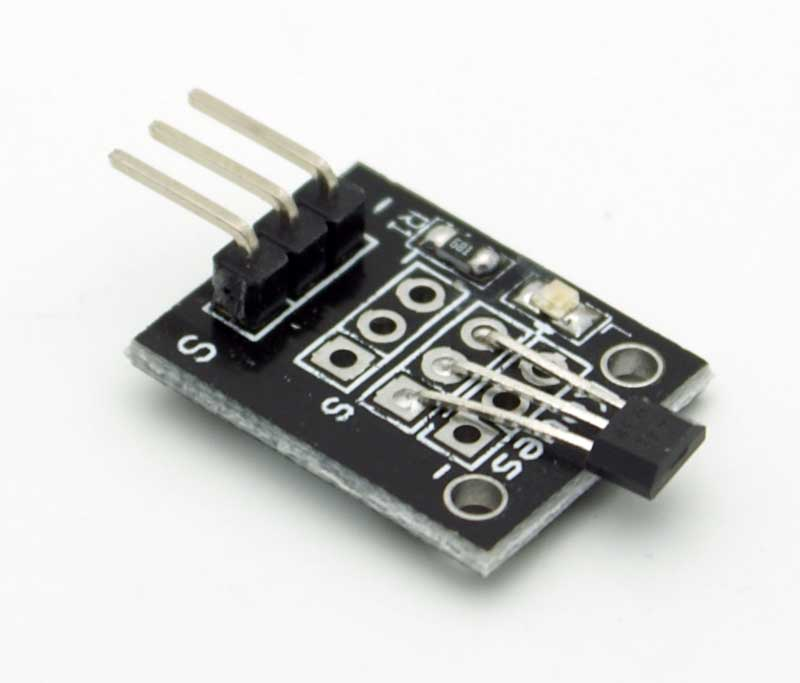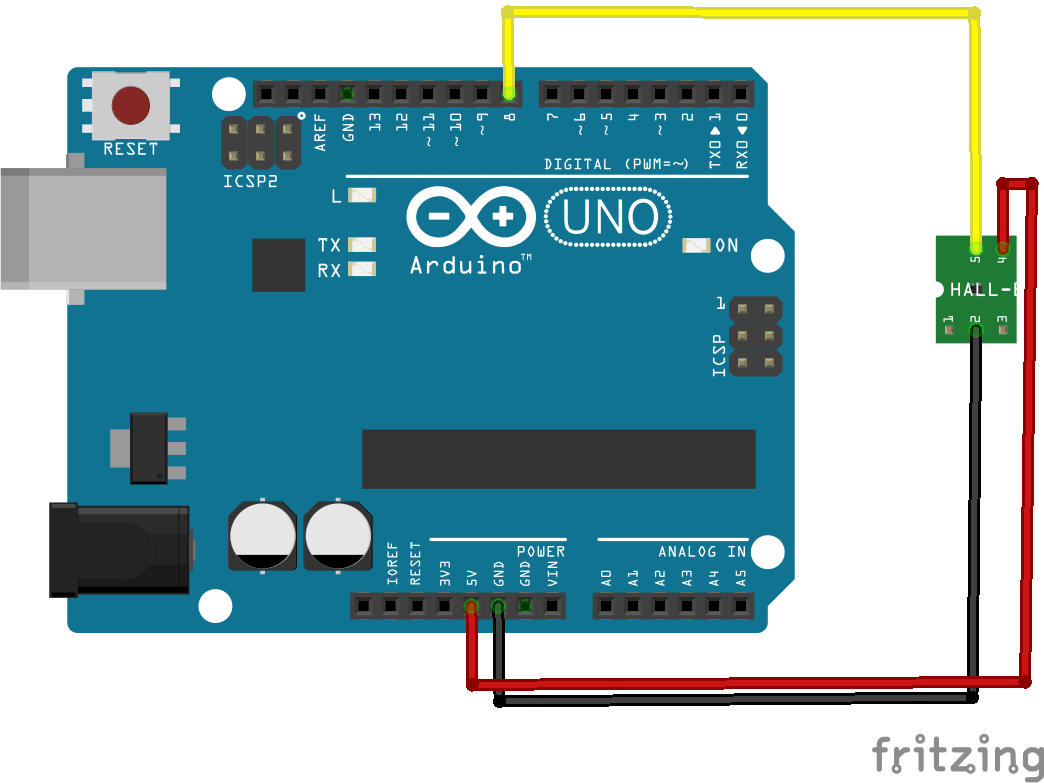# Arduino and hall effect sensor

A Hall effect sensor is a transducer that varies its output voltage in response to a magnetic field. Hall effect sensors are used for proximity switching, positioning, speed detection, and current sensing applications.

In its simplest form, the sensor operates as an analog transducer, directly returning a voltage. With a known magnetic field, its distance from the Hall plate can be determined. Using groups of sensors, the relative position of the magnet can be deduced.

Lets look at an example of a module that is popularly usedThe KY-003 is a Hall effect sensor. If no magnetic field is present, the signal line of the sensor is HIGH . If a magnetic field is presented to the sensor, the signal line goes LOW, at the same time the LED on the sensor lights up.

Schematics

Here is a breadboard layout created using parts in FritzingConnection

• Pin – is GND, connect to GND of the Arduino
• Middle pin is +5 v, connect to Arduino +5
• Pin S signal, connect to Arduino pin 8

You can use any Arduino input, we selected pin 8 for our example

Code

A fairly simple example here, there is a visual indication and also a debug option via the Serial Monitor in the Arduino IDE

 Source code```int Led = 13 ; // Arduino built in LED
int SENSOR = 8 ; // define the Hall magnetic sensor
int val ; // define numeric variables

void setup ()
{
Serial.begin(9600);
pinMode (Led, OUTPUT) ;    // define LED as output
pinMode (SENSOR, INPUT) ;  // define the Hall magnetic sensor line as input
}

void loop ()
{
if (val == LOW) // when the Hall sensor detects a magnetic field, Arduino LED lights up
{
digitalWrite (Led, HIGH);
Serial.println("Magnetic field detected");
}
else
{
digitalWrite (Led, LOW);
Serial.println("No magnetic field detected");
}
delay(1000);
}```

Run the following and put a magnet near the hall effect sensor, the LED should go on and if you have the serial monitor open you will see an appropriate message displayed